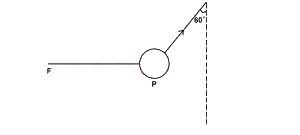# The body P shown in the diagram above is in equilibrium. If the mass Q of the body is 10kg, calculate the tension T in the string. (Take g = 10ms2)

Question 1

The body P shown in the diagram above is in equilibrium. If the mass Q of the body is 10kg, calculate the tension T in the string. (Take g = 10ms2)